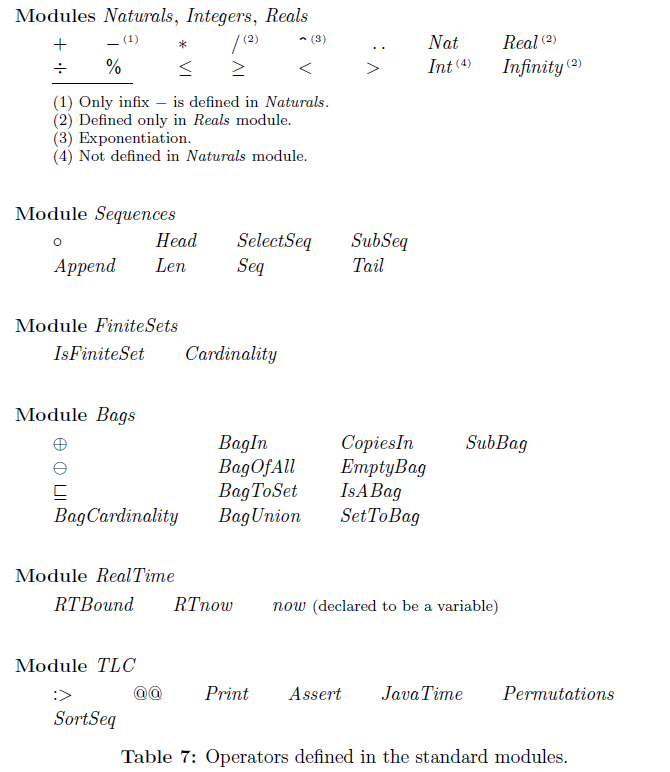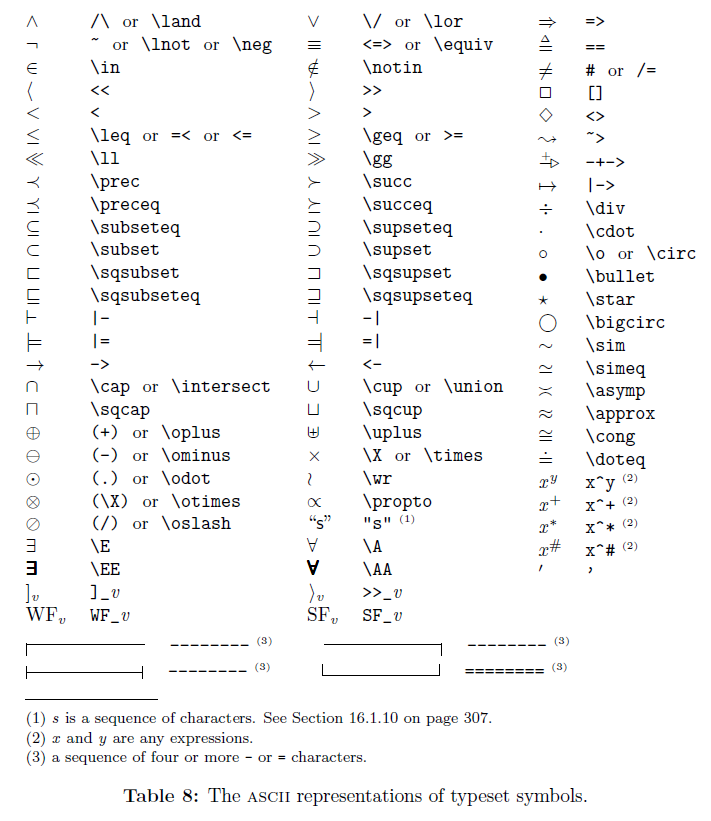TLA+ 相关笔记。

## 2. 个人笔记

Tips

 1 2 3 4 5 6 7 8 9  \documentclass[preview]{standalone} \begin{document} \tlatex \setboolean{shading}{true} \@x{}\bottombar\@xx{}% ...... ...... \@x{}\bottombar\@xx{}% \end{document} 

## 3. Operators of standard modules (P272)## 4. Typeset symbols of TLA+ (P273)Operator meaning
\* line comment
(* block comment
= is assignment in the variables block,
is the equality check in everywhere else
:= assignment
/= or # not equal
/\ and
\/ or
~ not
== define an operator
<<...>> Specify tuples and they are 1-indexed
\o Combine two sequences
\X Cartesian product operator: set1 \X set2 is the set of
all tuples t where t \in set1 and t \in set2.
{...} set
\in in set
\notin not in set
\subseteq is subset
\union set union
\intersect set intersection
S1 \ S2 the elements in S1 not in S2
\E exists
~\E not exists
\A all
~\A not all
P => Q If P is true, then Q must also be true
It’s equivalent to writing ~P \/ Q
P <=> Q Either both P and Q are true or both are false
It’s equivalent to writing (~P /\ ~Q) \/ (P /\ Q)
 ->, ->
the domain to a specific range. Use -> when you want
the set of functions that maps the domain to the range.

[] an invariant. []P means that P is true for all states
<> eventually. <>P means that for every possible behavior,
at least one state has P as true
~> leads to. P ~> Q implies that if P ever becomes true,
at some point afterwards Q must be true.
<>[] stays. <>[]P` says that at some point P becomes true
and then stays true.